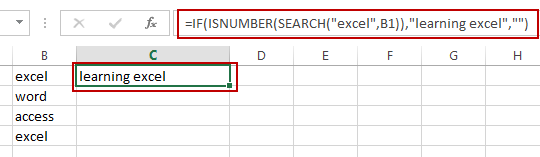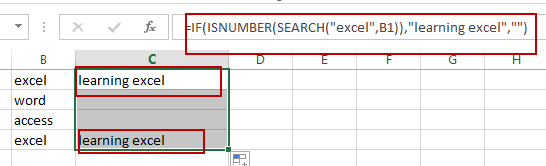# How to Return a Value If a Cell Contains a Specific Text in Excel

This post will guide you how to return a value if a cell contains a certain number or text string in Excel. How do I check if a Cell contains specific text and then return another specific text in another cell with a formula in Excel.

## Return Value If Cell Contains Certain Value

Assuming that you want to check if a given Cell such as B1 contains a text string “excel”, if True, returns another text string “learning excel” in Cell C1. How to achieve it. You can use a formula based on the IF function, the ISNUMBER function and the SEARCH function to achieve the result of return a value if Cell contains a specific value. Just like this:

`=IF(ISNUMBER(SEARCH("excel",B1)),"learning excel","")`

Type this formula into the formula box of cell C1, and then press Enter key in your keyboard.You will see that the text string “learning excel” will be returned in the Cell C1.

And if you want to check the range of cells B1:B4, you need to drag the AutoFill Handle down to other cells to apply this formula.Video: Return Value If Cell Contains Certain Value

### Related Functions

• Excel SEARCH function
The Excel SEARCH function returns the number of the starting location of a substring in a text string.The syntax of the SEARCH function is as below:= SEARCH  (find_text, within_text,[start_num])…
• Excel ISNUMBER function
The Excel ISNUMBER function returns TRUE if the value in a cell is a numeric value, otherwise it will return FALSE.The syntax of the ISNUMBER function is as below:= ISNUMBER (value)…
• Excel IF function
The Excel IF function perform a logical test to return one value if the condition is TRUE and return another value if the condition is FALSE.The syntax of the IF function is as below:= IF (condition, [true_value], [false_value])…
Related Posts

Filter or Extract with a Partial Match

Assume you have a table consisting of a few cells having few values, and you want to filter out the set of records with the partial match. You might take it easy and would prefer to manually filter out the ...

Extract all Partial Matches

Just assume that you have a range of data that contains a text string and you want to extract the few partial matches into another separate range of cells; then you might think that it's not a big deal; because ...

If Cell Contains Certain Text OR Equals Certain Text

IF cell equals certain text IF function is frequently used in Excel worksheet to return “true value” or “false value” based on the logical test result. If you want to test values to see if they equal certain text like ...

How to Extract Text between Two Text Strings in Excel

This post will guide you how to extract text between two given text strings in Excel. How do I get text string between two words with a formula in Excel. Extract Text between Two Text Strings Assuming that you have ...

Insert The File Path and Filename into Cell

This post will guide you how to insert the file path and filename into a cell in Excel. Or how to add a file path only in a specified cell with a formula in Excel. Or how to add the ...

Sort Cells by Specific word or words

This post will guide you how to sort cells or text values in a column based on a specific word even if the word is in the text string in the cell. How do I sort cells in a column ...

Highlight Rows

This post will teach you how to highlight rows in a table with conditional formatting in Excel. You will learn that how to change the color of the entire rows if the value of cells in a specified column meets ...

How to replace all characters after the first specific character

This post will guide you how to replace all characters after the first match of a specific character with a new text string in excel. How to replace all substrings after the first occurrence of the comma character with another ...

How to extract text after the second or nth specific character (space or comma)

In the previous post, we talked that how to extract text after the first occurrence of the comma character in excel. And this post explains that how to get a substring after the second or nth occurrence of the comma ...

How to extract text before the second or nth specific character (space or comma)

Before we talked that how to extract text before the first space or comma character in excel. And this post will guide you how to extract a substring before the second or nth specific character, such as: space or comma ...

Sidebar